August 2008: This page now requires Internet Explorer 6+ MathPlayer or Mozilla/Firefox/Netscape 7+.

CHAPTER IV
Differential Equations from an Elementary Viewpoint

Preface: Some thought experiments. We begin this chapter with some model contexts.
[Motion based model] Think of driving a car along a road. Your odometer is broken but your speedometer still works. You record only where the car is when you begin your trip and you make a table of the car's speed (velocity) at regular time intervals during the trip. [Or perhaps you can record the velocity at every time from the beginning to the end of the trip]. Based on this information do you think you could give your location approximately (or exactly) at any time during your travels? As a practical matter, if you have the time to repeat the trip this shouldn't be too difficult. With the information you recorded you can reproduce the trip with some accuracy. This time use a car with an odometer and let someone else do the driving according to the recorded velocities while you record your position.

For an hour trip this might be more time than you want to spend on this. With a little thought and few arithmetic calculations, you should become up with a reasonable estimate of where you were at the same points in time where you recorded the velocities.

 t minutes v(t) miles/hour 0 30 15 60 30 60 45 50 60 40
Click here to see a position function that matches these instananeous velociteis.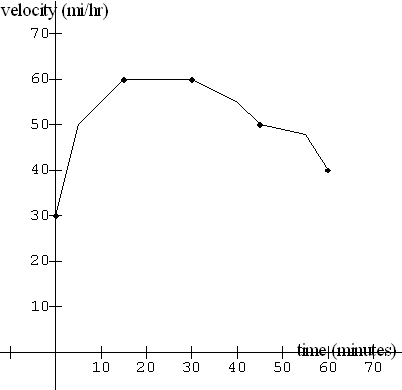Graph of Velocities
[Motion based model] Next suppose you are driving on a highway through a major city. (Los Angeles, Chicago, New Orleans, Miami, or New York will do). You are trying to explain to your passenger why you are travelling at your current speed. What would you include in your explanation? The time of day (6 a.m. or 6 p.m.)? Or the position in the road ( before a major interchange, on a stretch where construction is under way, or on a section where two additional lanes have been added)? Or perhaps the speed at which other vehicles are travelling ( you are travelling up a hill behind two large trucks)? Based on this information can you tell your passenger where you will be in the next few seconds, minutes, hours? How accurate will your predictions be?
 time\ Mile marker           on highway 5 8 10 12 6:00 60 55 50 60 8:30 40 40 25 50 11:00 50 50 45 55 15:00 55 50 45 60 18:00 40 30 35 40 23:00 60 60 50 60

[Production based model.] Think of manufacturing a very desirable product, "good stuff." As your production increased you forgot to keep track of your accumulated costs but you did keep your receipts showing you the marginal rates you were charged for materials and labor. Based on this information, do you think you could give your accumulated costs approximately (or exactly) at any stage of the production? As a practical matter, this is what accountants do in reviewing the accumulation of costs. With the information you have retained you should be able to reproduce the accumulation of costs with great accuracy.
 Units  produced Marginal cost \$/ unit 0 to 5 4.00 5 to 12 3.50 12 to 20 3.25 20 to 40 2.00 40 to 50 3.00
Click here to see an accumulated cost function that matches these marginal costs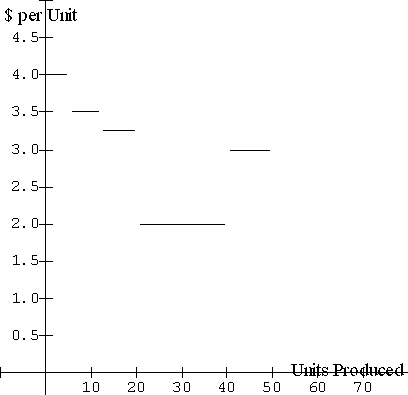Graph of Marginal Cost
per Unit Produced

[The Dart Model] Suppose you are throwing a dart at a region in the plane and measuring a random variable X determined by the position of the dart. X has values only between 0 and 10. Unfortunately you are not given the distribution function of X. [Recall that for any real number A between 0 and 10 the distribution function F, would give you F(A), the probability that the value of X is less than or equal to A.]
Instead you have been given a graph, an algebraic expression and a table for the probability density function of X. [Recall that  for any real number A between 0 and 10 the probability density function of X, f, gives you  f(A), the derivative of F at A, which is approximately the ratio of the probability of the random variable X being in an interval [A, A+h] to the length of that interval, h.]
Based on this information do you think you could estimate the probability that the value of X being less 5? Recognizing that F(0) = 0 and F(10) = 1, could you estimate F(A) for any A between 0 and 10? As a practical matter, you might break the interval into10 pieces of equal length and then estimate the probability of X being in each of those interval from the density of X at some point in that interval. Then for A, just add up the probability estimates for X being in an interval that has values less than or equal to A.
 A f (A) 0.5 .05 1.5 .13 2.5 .11 3.5 .15 4.5 .19 5.5 .17 6.5 .09 7.5 .07 8.5 .03 9.5 .01
Click here to see a probability distribution function for X that matches these probability densities.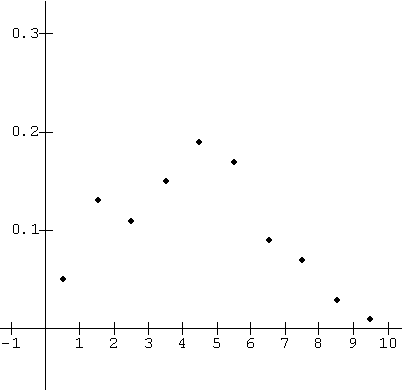Graph of data for f(A)
The Probability Density Function for X

[The Treasure Hunt] Here's another context. You are on a treasure hunt. Besides telling you where to start and which way to go, the directions tell you the speed and direction you should travel and how long you should follow that instruction. Could you find the treasure? Would you be able to tell where you were at any time during this trip?
 The Treasure Hunt Directions  Start at (20,10) on the map. Head north at 3 feet per second for exactly 20 seconds.  Now turn east and proceed at 2 feet per second for 10 seconds. At this point head south at 4 feet per second for 5 seconds. Turn and proceed due west at one foot per second for 8 seconds. You should be at the treasure now! Good luck.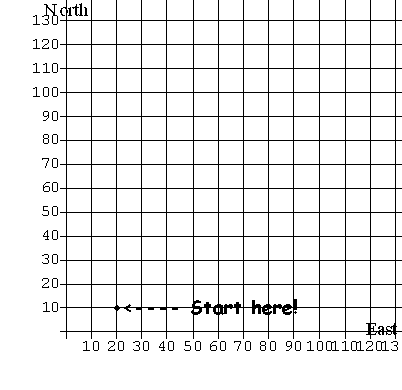Click on this map
to find the treasure.

Though these illustrations may seem a little artificial, the contexts reflect many real circumstances. Often we know something about the rate at which some measured quantity (e.g., rain, snow, population of owls or fruit flies, people infected with a virus, chemical, or money) is changing. Using these rates (i) we would like to know the quantity present at certain times, i.e., when t = a,  find f (a); or (ii) we would like to predict when the quantity will reach certain levels (either desirable or undesirable), i.e., given c, find so that when t = b,  f(b) = c. Here are two key questions:
1. How does the rate of change for quantities control the actual amounts of those quantities as they change? and
2. What controls the rates of change?
Scientific investigators and engineers look for relations that determine rates of change in real contexts along with specific measurements from which the information can be extended, by interpolation or extrapolation.

The mathematical investigation of rates using calculus is the defining objective for the study of differential equations. This is one of the most important and, therefore, most studied parts of mathematics. It will be a major theme for the remainder of this book. You should note that this is not our first discussion of rates and related contexts. In our study of implicit differentiation and problems with related rates we encountered many equations involving derivatives of varying complexity. Often the derivative in these studies depended on both the x and y variables.

For example, implicitly differentiating the equation y3 = x2 with respect to x leads to the derivative equation, dy/dx = 2x/3y2. Differentiating the same equation with respect to the variable t (which is not expressed in the equation) leads to the derivative equation 3y2 dy/dt = 2x dx/dt. [In fact, we could also express these last two situations with one equation using differentials: 3y2 dy = 2x dx.]

In this chapter we begin our examination of differential equations and some of the basic solution concepts for these equations. In doing this we will use our previous work on the derivative (and differentials) to explore these concepts visually, numerically, and symbolically. Our purpose is to develop some systematic methods (a calculus) for solving differential equations. As we proceed through the chapter we will follow a recurrent path of

1. examining cases that are simple and known with a new tool for understanding a differential equation,
2. describing how the tool is managed with some generality, and
3. applying the tool to cases where previous techniques are not as successful.

IV. A. Differential Equations. Basic Concepts and Definitions.

A differential equation (D.E.) is an equation involving derivatives in its statement. Here are some examples:

 (i) dy/dx = x 2 (ii) y' - x + x 2 = 3xy (iii) y' + xy = (y') 2 (iv) f ''(x) = 5 (v) p''(x) +xp'(x) + p(x) = 0 (vi) Dt f(t) + t f(t) = 3 (vii) y'' + y' = 5 + y''' (viii) du/dt + dv/dt = 5
A differential equation involving only first derivatives is called a first order differential equation. Examples (i)-(iii), (vi), and (viii) are all first order differential equations. When no higher than the second derivative is involved in the equation it is called a second order differential equation. Examples (iv) and (v) are second order differential equations. Higher order differential equations involve higher order derivatives. As you might guess at this point, equation (vii) is a third order differential equation.

In some situations a differential equation may be expressed using differentials! For example, the equation dy/dx = x2/y might be expressed as dy = x2/y dx or as y dy = x2 dx.

Interpretation: We have seen that a derivative can be interpreted dynamically as a rate of change (of various orders) or geometrically as the slope of a tangent line. Following the dynamic view, a differential equation can be interpreted as a relation between measured quantities and their rates of change. Understanding these relations is at the heart of most questions in the sciences. A scientist studies not only what things are but also how things change. An understanding of change allows scientists (and engineers) to predict and thereby control different aspects of our world. This is easy to believe in the physical, chemical, and biological science, but it is increasingly true as well in such disciplines as economics, management, geography, and other social sciences. You can find contexts for using differential equations almost anywhere you look.

Differential equations for motion were among the early motivations for developing the calculus. Today the need to analyze and control change in complex situations is a major reason why the study of calculus and differential equations is considered so important. These equations are used to study everything:

• from the forces in the smallest subatomic particles, to the motion of space craft,
• from the growth of small cell and insect populations to the complex interrelations of environmental systems,
• from the effect of a sudden cooling on a hot summer day to the global effects of a hole in the ozone layer of the atmosphere, and
• from the profitability of a single business decision to the effect of major fiscal and monetary policy changes on national and international economics.
Be it mechanics, heat, sound, light, or the price of butter in Burbank, whatever the subject, there is usually one or more differential equations useful in its study. It is should be no surprise then that so many disciplines require their students learn calculus.

 I am sure you know what it means to solve an equation in algebra. It means to find all those values chosen from a given set of possible solutions that will make the given equation true. So, for example, 2 and -2 are the solutions from the set of integers for the equation x2 = 4. Similarly, the equation sin(t) = 0 has an infinite set of solutions when we allowing real numbers as possible solutions, namely {`t = 2 pi k` where k is any integer}, but this same equation has only one solution that is a rational number, namely 0.
A solution to a differential equation with one dependent variable is a differentiable (hence, continuous) function which satisfies the differential equation on an interval domain.
Thus y = x 3 / 3 + 5 is a solution to the differential equation dy/dx = x 2. This solution is not unique, since y = x 3/3 - 9 will satisfy the same differential equation.

As a second example you can check that  `y = sqrt{25 - x^2}` on the interval (-5,5) and  `y = sqrt{25 - x^2}`on the interval (-4,4) are both solutions to the equation dy/dx = -x/y. With these examples we can identify two key issues in the study of differential equations.

Issue I: When does a differential equation have a solution?
Issue II: How unique is the solution of a given differential equation?
We observe as a first response to these issues that it is easier to check whether or not a function is a solution to a given differential equation than it is to find a solution.

The general solution to a differential equation is the family of all functions that are solutions to a given differential equation. For example, the general solution to the differential equation dy/dx = x 2 is the family of functions of the form y = x3 / 3 + C where C is a constant. [This statement should seem plausible at this stage. It will be justified in Section IV.B.1.] The general solution to the equation dy/dx = -x/y is the family of functions of the form `y = +-sqrt{a^2 - x^2}` on the interval (-a, a) where a>0 is a constant. [This statement will be justified in Chapter VII.]

We can now identify a third key issue in the study of differential equations, one that focuses not on existence or uniqueness of a solution, but on the ability to describe a solution in familiar terms.
Issue III: Is it possible to describe the general solution to a differential equation as some elementary function?
This is not always possible. For example, there is no description possible for the differential equation dy/dx = sin(x2) as an elementary function.[This result is not easy to justify.] For that reason we are also interested in describing the general solution graphically and estimating values for solutions numerically.

Conventions in Language and Notation:
When the differential equation has the form y' = P(x) where P is a function of x only, the general solution is also called the indefinite integral of P(x) or the anti-derivative of P(x) and is denoted
`int P(x) dx`.
[N.B. In this situation the variable y does not control the derivative, y'.]

This is usually read as "the (indefinite) integral of P(x) with respect to x " or more simply "the (indefinite) integral of P." [The word "indefinite" is frequently omitted.]

P(x) is called the "integrand" of the indefinite integral. [The significance and utility of this notation will become more apparent later.] The process of finding the general solution in this case is called "integration."

Continuing the previous example, writing   `int x^2 dx = 1/3 x^3 + C` is understood to mean that the indefinite integral of the function given by the rule x 2 with respect to variable x is the family of functions of the form
`1/3 x^3 + C`  where `C`  is a constant. [This follows the conventional abuse of notation: name a function by its rule and then announce the controlling variable for the rule.] In the general solution, the term "`C`" is described as a constant of integration.
It might be more correct to write `int x^2 dx =` {`1/3 x^3 + C` : where C is any constant }.
The braces here indicate that we are describing a family of functions as opposed to one generic member of the family. This notation is not conventional and we will continue in the text to ignore this overly refined view. Remember that in conventional notation there is some ambiguity in representing the family of functions by a single generic member.

As the remarks in the preface to this chapter indicated, knowing where you start on a trip determines in part where you will be subsequently. Often a differential equation has one or more additional conditions which a solution must satisfy as well, such as y(0) = 7 or y(3) = 4. Additional conditions often arise in applications from information given about the beginning of a situation ("initial conditions") or conditions that must be satisfied at the beginning and end of the process ("boundary conditions"). Such conditions restrict the selection of solutions to only a few particular members from the family of all solutions. A particular (or special) solution to a differential equation is a solution that satisfies any such additional conditions.

Continuing with our example of the differential equation dy/dx = x 2, we suppose that the general solution is y = x 3/3 + C. If we use the initial condition y(0)=7 with this situation, it must be that

7 = (0) 3/3 + C, so C = 7.
Thus the particular solution to this differential equation with the given initial condition is y = x 3/3 + 7. [This is easily checked by finding the derivative, dy/dx, and evaluating y when x = 0.]

Looking at the differential equation dy/dx = -x/y, we suppose that the general solution is  `y = +-sqrt{a^2 - x^2}` where a is a constant. If we use the condition y(3)=4 with this situation, it must be that  `4 = y(3) = +sqrt{a^2 - 3^2}` , so  `16 = a^2 - 9` and `a^2 = 25` and therefore
`y = +sqrt{25 - x^2}` is the particular solution to this differential equation with the given condition.

Notice that in each of these examples there is only one function that will satisfy both the differential equation and the given initial condition, i.e., the solution in these special situations is unique.

Key Words: Look the following words up in a dictionary. Compare the dictionary definition(s) to the use of the word in this section.

 differential equation boundary solution antiderivative particular condition integral general initial indefinite unique

PROBLEMS IV.A

1. For each differential equation determine whether or not the given function is a solution.

 a. dy/dx = 3x 2 - 2x + 5; y = x 3 - x 2 + 5x - 8. b. y' + 2y = x 2 + 3x + 5; y = x 2 + x + 4. c. dy = 12x cos(x 2) dx; y = 6 sin (x 2) + 25 d. y dx + x dy = 4x 3 dx;. y = x 3 e. y''= 12x 2 - sin(x) + 2; y = x 4 + sin(x) + x 2 + 3x + 5 f. P''(t) + P(t) = 0; P(t) = 5 sin(t) - 10 cos(t) g. d2 z/du2 - 9z = 0; z = e3u h. g'(t)= 2/t + 3e3t ; g(t) = 2 ln(t)+ e3t
2.The following table shows the velocity of a car during a trip on a rural highway recorded at 30 second intervals. Based on this information estimate the answer to the following questions. Explain your reasoning briefly.
 time (min) 0 0.5 1 1.5 2 2.5 3 3.5 4 4.5 5 5.5 6 velocity (mi/h) 0 30 25 30 10 -15 -25 -10 25 40 50 50 30
a. How far was the car from its starting point after 3 minutes and after six minutes?
b. How many miles did the odometer change after 3 minutes and after six minutes?
c. When during the trip did the car pass a point on the highway 3 miles from the starting point?
d. When did the car turn around and head back to the starting point?
e. The car turned around because the driver wanted to check on the name on a mailbox they had passed. Approximately where was the mailbox located?

3. In the figure below is the graph of the rate at which water was used during a twenty four hour period in the town of USH. Based on the graph draw a sketch of a graph showing the amount of water used as a function of time during the same period. Estimate the amount of water consumed during the twenty four hour period.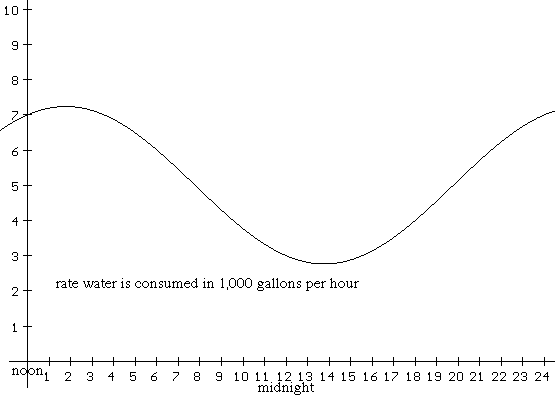4. Suppose that the general solution to the differential equation dy/dx = 3x 2 + 5 is given by y = x 3 + 5x + C where C is a constant. Determine the value of C that will give a solution to this differential equation with the given condition.

(a) y(0) = 7 (b) y(0) = -4
(c) y(1) = 7 (d) y(1) = -4

5. Suppose that the general solution to the differential equation y''+ y = 0 is given by y = Asin(x) + Bcos(x) where A and B are constants. Determine the values of A and B that will give a solution to this differential equation with the given conditions.

(a) y(0) = 5 ,  y'(0) = 6   (b) y(0) = -3 , y'(0) = 4
(c) y(0) = 2 , y(p/2)= 3   (d) y(0) = -2 , y(p/2)= 5

In problems 6 and 7, do not try to solve the differential equations. Without knowing explicitly what y is as a function of x, but using knowledge of its derivative from the differential equation, there is still much you can say about y in these problems..

6. Assume y is a solution to the differential equation dy/dx= x/(x 2 + 1) with y(0)=2.
(a) Using just the given information, find any local extreme points for y and discuss the graph of y, including the issue of concavity.
(b) Using the differential, estimate y(1) and y(-1).
(c) Change the initial condition to y(0) = 3 and then y(0)= 1. How will these different conditions affect the graph of y? How do they affect the estimates for y(1) and y(2) made in part b?

7. Assume y is a solution to the differential equation dy/dx= y - 1 with y(0)=2 and y(x) >1 for all x.
(a) Using just the given information, discuss the graph of y including the issue of concavity.
(b) Using the differential, estimate y(1/2) and y(-1/2).
(c) Suppose that y(x) <1 for all x and y(0) = 0. How will these different conditions affect the graph of y? How do they affect the estimates for y(1/2) and y(-1/2) in part b?

8. Match each indefinite integral with the family of functions that best describes it.

 (a) ∫ x3 dx (i) -x2/6+C (b) ∫ 3x dx (ii) (3/4)x4/3+C (c) ∫ x-3 dx (iii) (1/4)x4 +C (d) ∫ x1/3 dx (iv) (3/2)x2+C (e) ∫ -x/3 dx (v) -x-2/2+C (vi) 3x4/3+C
9. Assume y is a solution to the differential equation dy/dx= (y-1)(x 2 -1).
(a) Assume that y(0)=2. Using the differential, estimate y(1/2) and y(-1/2).
(b) Change the initial condition on y to y(0) = 3 and then y(0)= 1. How will these different conditions affect your estimates?
(c) Which of the following graphs are likely to be the graph of a solution for this differential equation .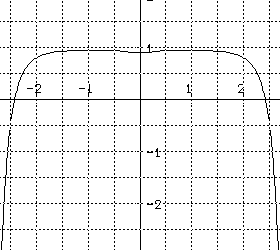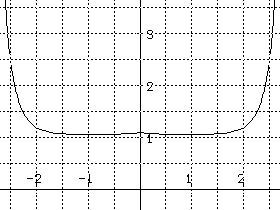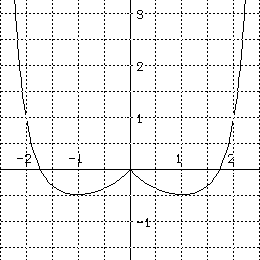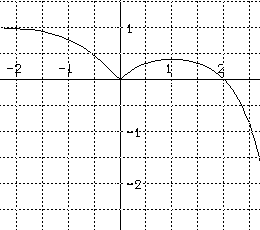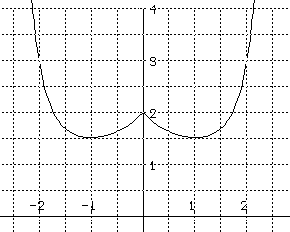10. Match the following statements with the differential equation that best represents the situation described.

(a) As the runner approached the end of the race, she slowed her rate steadily so that when she crossed the finish line at 20 seconds she was travelling at 7 meters per second.
(i) dr/dt = -7t+ 20 (ii) r'(t)=-kt + 7(t-20) (iii) dr/dt = -k(t-20) + 7

(b) The population of cells had a biomass of 30 milligrams when the experiment began. The biomass grew a rate proportional to the mass present.
(i) P'(t) = k t (ii) P'(t) = k P(t) (iii)P'(t)= k (30 + P(t))

(c) The temperature of the coffee was initially 373 degrees Kelvin while the room was only about 333 degrees.. The coffee's temperature decreased at a rate proportional to the difference between its temperature and that of the room.
(i) dT/dt = -k (T-333) (ii) dT/dt = -k ( 373t - 333) (iii) dT/dt = -k/(t-333)

(d) After 10 minutes the student was learning the material more slowly as time went on, so that his rate of learning was then decreasing inversely with the square of the time he had spent reviewing.
(i) dL/dt = k (t +10)2 (ii) DL(t) = k/t2 (iii) L'(t) = k/(t+10)2

(e) The profits from the investment were increasing more slowly as time went on so that the rate appeared to decrease inversely proportionately with the profits at any given time.
(i) P'(t)=k - P(t) (ii) DP(t) = k/t (iii) dP/dt = k/P

(f) The marginal revenues from the production of more than 100 faucets decrease proportionately with the number of additional faucets produced.
(i) dR/dx = -kx (ii) DR(x) = -k(x+100) (iii) R' = -k(x-100)

11. Which of the following equations mean the same thing? Explain your response.

4t3 dt = t 4 + C; 4x3 dx = x 4 + C;
D( t4 + C) = 4t3; D(x4 + C) = 4x3

12. Use the interpretation of the derivative as the velocity of a moving object to explain the following statement: If two functions have the same derivative at every point then the difference between the values of the functions at every point will be the same number.

13. Describe three contexts where the rate at which something is used is monitored to predict how much will be used in the future. Discuss how do these predictions affect planning?

14. The following table gives the speed of cars on a rural highway at the given time of day and the mile maker.

 \ time  mile marker 5 a.m. 8 a.m. 11 a.m. 10 mi. 55 mph 40 mph 50 mph 20 mi. 30 mph 20 mph 30 mph 30 mi. 40 mph 25 mph 30 mph
Based on this table estimate the time it takes to travel from mile 10 to mile 40 at 5 a.m., at 8 a.m., and at 11 a.m. Discuss briefly the assumptions you made in making your estimates.

15. Sometimes it is possible to show that a differential equation cannot be solved by functions of designated type. For each of the following differential equations do not try to find a solution. Based on the equation explain why there can not be any polynomial function that would satisfy the equation.

a) f '(x) = 5 f(x) and f(0) = 2. [Hint: Find a formula for f (n)(x). Show that f (n) (0) is not 0 for any nth order derivative of f.]
b) f ''(x) = -5 f(x) and f(0) = 2.
c) dy/dx = 1/(1 + x2) and y(0) = 2.

16. Suppose X is a random variable with range [0,10]. Give a differential equation that shows the relation of the probability density function f (x) and the distribution function F(x) for X. Explain briefly why the boundary conditions for this differential equation are F(0) = 0 and F(10)=1. Generalize this situation to a random variable with range [A,B].